# Numeric Precision in SAS Software

### How SAS Stores Numeric Values

To store numbers of large magnitude and to perform computations that require many digits of precision to the right of the decimal point, SAS stores all numeric values using floating-point , or real binary, representation. Floating-point representation is an implementation of what is generally known as scientific notation, in which values are represented as numbers between 0 and 1 times a power of 10. The following is an example of a number in scientific notation: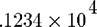Numbers in scientific notation are comprised of the following parts :

• The base is the number raised to a power; in this example, the base is 10.

• The mantissa is the number multiplied by the base; in this example, the mantissa is .1234.

• The exponent is the power to which the base is raised; in this example, the exponent is 4.

Floating-point representation is a form of scientific notation, except that on most operating systems the base is not 10, but is either 2 or 16. The following table summarizes various representations of floating-point numbers that are stored in 8 bytes.

Table 5.7: Summary of Floating-Point Numbers Stored in 8 Bytes

Representation

Base

Exponent Bits

Maximum Mantissa Bits

IBM mainframe

16

7

56

IEEE

2

11

52

SAS allows for truncated floating-point numbers via the LENGTH statement, which reduces the number of mantissa bits. For more information on the effects of truncated lengths, see "Storing Numbers with Less Precision" on page 95.

### Troubleshooting Problems Regarding Floating-Point Representation

#### Overview

In most situations, the way that SAS stores numeric values does not affect you as a user . However, floating-point representation can account for anomalies you might notice in SAS program behavior. The following sections identify the types of problems that can occur in various operating environments and how you can anticipate and avoid them.

#### Floating-Point Representation on IBM Mainframes

SAS for z/OS uses the traditional IBM mainframe floating-point representation as follows :

` SEEEEEEE MMMMMMMM MMMMMMMM MMMMMMMM    byte 1   byte 2   byte 3   byte 4    MMMMMMMM MMMMMMMM MMMMMMMM MMMMMMMM    byte 5   byte 6   byte 7   byte 8 `

This representation corresponds to bytes of data with each character being 1 bit, as follows:

• The S in byte 1 is the sign bit of the number. A value of 0 in the sign bit is used to represent positive numbers.

• The seven E characters in byte 1 represent a binary integer known as the characteristic . The characteristic represents a signed exponent and is obtained byadding the bias to the actual exponent. The bias is an offset used to allow for both negative and positive exponents with the bias representing 0. If a bias is not used, an additional sign bit for the exponent must be allocated. For example, if a system employs a bias of 64, a characteristic with the value 66 represents an exponent of +2, while a characteristic of 61 represents an exponent of ˆ’ 3.

• The remaining M characters in bytes 2 through 8 represent the bits of the mantissa. There is an implied radix point before the leftmost bit of the mantissa; therefore, the mantissa is always less than 1. The term radix point is used instead of decimal point because decimal point implies that you are working with decimal (base 10) numbers, which might not be the case. The radix point can be thought of as the generic form of decimal point.

The exponent has a base associated with it. Do not confuse this with the base in which the exponent is represented; the exponent is always represented in binary, but the exponent is used to determine how many times the base should be multiplied by the mantissa. In the case of the IBM mainframes, the exponent's base is 16. For other machines, it is commonly either 2 or 16.

Each bit in the mantissa represents a fraction whose numerator is 1 and whose denominator is a power of 2. For example, the leftmost bit in byte 2 represents ( ½) 1 , the next bit represents ( ½) 2 , and so on. In other words, the mantissa is the sum ofa series of fractions such as ½, ¼, , and so on. Therefore, for any floating-point number to be represented exactly, you must be able to express it as the previously mentioned sum. For example, 100 is represented as the following expression: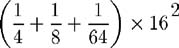To illustrate how the above expression is obtained, two examples follow. The first example is in base 10. The value 100 is represented as follows:

` 100. `

The period in this number is the radix point. The mantissa must be less than 1; therefore, you normalize this value by shifting the radix point three places to the right, which produces the following value: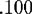Because the radix point is shifted three places to the right, 3 is the exponent: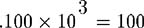The second example is in base 16. In hexadecimal notation,100 (base 10) is written as follows: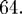Shifting the radix point two places to the left produses the following value: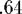Shifting the radix point also produces an exponent of 2, as in: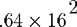The binary value of this number is .01100100 , which can be represented in the following expression: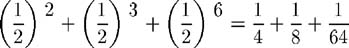In this example, the exponent is 2. To represent the exponent, you add the bias of 64 to the exponent. The hexadecimal representation of the resulting value, 66, is 42. The binary representation is as follows:

` 01000010 01100100 00000000 00000000   00000000 00000000 00000000 00000000 `

#### Floating-Point Representation on OpenVMS

On OpenVMS, SAS stores numeric values in the D-floating format, which has the following scheme:

` MMMMMMMM MMMMMMMM MMMMMMMM MMMMMMMM    byte 8   byte 7   byte 6   byte 5    MMMMMMMM MMMMMMMM SEEEEEEE EMMMMMMM    byte 4   byte 3   byte 2   byte 1 `

In D-floating format, the exponent is 8 bits instead of 7, but uses base 2 instead of base 16 and a bias of 128, which means the magnitude of the D-floating format is not as great as the magnitude of the IBM representation. The mantissa of the D-floating format is, physically, 55 bits. However, all floating-point values under OpenVMS are normalized, which means it is guaranteed that the high-order bit will always be 1. Because of this guarantee, there is no need to physically represent the high-order bit in the mantissa; therefore, the high-order bit is hidden.

For example, the decimal value 100 represented in binary is as follows:

` 01100100. `

This value can be normalized by shifting the radix point as follows:

` 0.1100100 `

Because the radix was shifted to the left seven places, the exponent, 7 plus the bias of 128, is 135. Represented in binary, the number is as follows:

` 10000111 `

To represent the mantissa, subtract the hidden bit from the fraction field:

` .100100 `

You can combine the sign (0), the exponent, and the mantissa to produce the D-floating format:

` MMMMMMMM MMMMMMMM MMMMMMMM MMMMMMMM   00000000 00000000 00000000 00000000   MMMMMMMM MMMMMMMM SEEEEEEE EMMMMMMM   00000000 00000000 01000011 11001000 `

#### Floating-Point Representation Using the IEEE Standard

The Institute of Electrical and Electronic Engineers (IEEE) representation is used by many operating systems, including Windows and UNIX. The IEEE representation uses an 11-bit exponent with a base of 2 and bias of 1023, which means that it has much greater magnitude than the IBM mainframe representation, but sometimes at the expense of 3 bits less in the mantissa. The value of 1 represented by the IEEE standard is as follows:

` 3F F0 00 00 00 00 00 00 `

#### Precision Versus Magnitude

As discussed in previous sections, floating-point representation allows for numbers of very large magnitude (numbers such as 2 to the 30th power) and high degrees of precision (many digits to the right of the decimal place). However, operating systems differ on how much precision and how much magnitude they allow.

In "How SAS Stores Numeric Values" on page 90, you can see that the number of exponent bits and mantissa bits varies. The more bits that are reserved for the mantissa, the more precise the number; the more bits that are reserved for the exponent, the greater the magnitude the number can have.

Whether precision or magnitude is more important depends on the characteristics of your data. For example, if you are working with physics applications, very large numbers may be needed, and magnitude is probably more important. However, if you are working with banking applications, where every digit is important but the number of digits is not great, then precision is more important. Most often, SAS applications need a moderate amount of both precision and magnitude, which is sufficiently provided by floating-point representation.

#### Computational Considerations of Fractions

Regardless of how much precision is available, there is still the problem that some numbers cannot be represented exactly. In the decimal number system, the fraction 1/3 cannot be represented exactly in decimal notation. Likewise, most decimal fractions (for example, .1) cannot be represented exactly in base 2 or base 16 numbering systems. This is the principle reason for difficulty in storing fractional numbers in floating-point representation.

Consider the IBM mainframe representation of .1:

` 40 19 99 99 99 99 99 99 `

Notice the trailing 9 digit, similar to the trailing 3 digit in the attempted decimal representation of 1/3 (.3333 ). This lack of precision is aggravated by arithmetic operations. Consider what would happen if you added the decimal representation of 1/3 several times. When you add .33333 to .99999 , the theoretical answer is 1.33333 2, but in practice, this answer is not possible. The sums become imprecise as the values continue.

Likewise, the same process happens when the following DATA step is executed:

` data _null_;      do i=-1 to 1 by .1;        if i=0 then put 'AT ZERO';      end;   run; `

The AT ZERO message in the DATA step is never printed because the accumulation of the imprecise number introduces enough error that the exact value of 0 is never encountered . The number is close, but never exactly 0. This problem is easily resolved by explicitly rounding with each iteration, as the following statements illustrate:

` data _null_;      i=-1;      do while(i<=1);         i=round(i+.1,.001);         if i=0 then put 'AT ZERO';      end;   run; `

#### Numeric Comparison Considerations

As discussed in "Computational Considerations of Fractions" on page 94, imprecision can cause problems with computations. Imprecision can also cause problems with comparisons. Consider the following example in which the PUT statement is not executed:

` data _null_;      x=1/3;      if x=.33333 then put 'MATCH';   run; `

However, if you add the ROUND function, as in the following example, the PUT statement is executed:

` data _null_;      x=1/3;      if round(x,.00001)=.33333 then put 'MATCH';   run; `

In general, if you are doing comparisons with fractional values, it is good practice to use the ROUND function.

#### Storing Numbers with Less Precision

As discussed in "How SAS Stores Numeric Values" on page 90, SAS allows for numeric values to be stored on disk with less than full precision. Use the LENGTH statement to dictate the number of bytes that are used to store the floating-point number. Use the LENGTH statement carefully to avoid significant data loss.

For example, the IBM mainframe representation uses 8 bytes for full precision, but you can store as few as 2 bytes on disk. The value 1 is represented as 41 10 00 00 00 00 00 00 in 8 bytes. In 2 bytes, it would be truncated to 41 10. You still have the full range of magnitude because the exponent remains intact; there are simply fewer digits involved. A decrease in the number of digits means either fewer digits to the right of the decimal place or fewer digits to the left of the decimal place before trailing zeroes must be used.

For example, consider the number 1234567890, which would be .1234567890 to the 10th power of 10 (in base 10). If you have only five digits of precision, the number becomes 123460000 (rounding up). Note that this is the case regardless of the power of 10 that is used (.12346, 12.346, .0000012346, and so on).

The only reason to truncate length by using the LENGTH statement is to save disk space. All values are expanded to full size to perform computations in DATA and PROC steps. In addition, you must be careful in your choice of lengths, as the previous discussion shows.

Consider a length of 2 bytes on an IBM mainframe system. This value allows for 1 byte to store the exponent and sign, and 1 byte for the mantissa. The largest value that can be stored in 1 byte is 255. Therefore, if the exponent is 0 (meaning 16 to the 0th power, or 1 multiplied by the mantissa), then the largest integer that can be stored with complete certainty is 255. However, some larger integers can be stored because they are multiples of 16. For example, consider the 8-byte representation of the numbers 256 to 272 in the following table:

Table 5.8: Representation of the Numbers 256 to 272 in Eight Bytes

Value

Sign/Exp

Mantissa 1

Mantissa 2-7

Considerations

256

43

10

000000000000

trailing zeros; multiple of 16

257

43

10

100000000000

extra byte needed

258

43

10

200000000000

259

43

10

300000000000

.

.

.

271

43

10

F00000000000

272

43

11

000000000000

trailing zeros; multiple of 16

The numbers from 257 to 271 cannot be stored exactly in the first 2 bytes; a third byte is needed to store the number precisely. As a result, the following code produces misleading results:

` data temp;      length x 2;      x=257;      y1=x+1;   run;   data _null_;      set temp;      if x=257 then put 'FOUND';      y2=x+1;   run; `

The PUT statement is never executed because the value of X is actually 256 (the value 257 truncated to 2 bytes). Recall that 256 is stored in 2 bytes as 4310, but 257 is also stored in 2 bytes as 4310, with the third byte of 10 truncated.

You receive no warning that the value of 257 is truncated in the first DATA step. Note, however, that Y1 has the value 258 because the values of X are kept in full, 8-byte floating-point representation in the program data vector. The value is only truncated when stored in a SAS data set. Y2 has the value 257, because X is truncated before the number is read into the program data vector.

 Caution Do not use the LENGTH statement if your variable values are not integers. Fractional numbers lose precision if truncated. Also, use the LENGTH statement to truncate values only when disk space is limited. Refer to the length table in the SAS documentation for your operating environment for maximum values.

#### Truncating Numbers and Making Comparisons

The TRUNC function truncates a number to a requested length and then expands the number back to full length. The truncation and subsequent expansion duplicate the effect of storing numbers in less than full length and then reading them. For example, if the variable

` x=1/3; `

is stored with a length of 3, then the following comparison is not true:

` if x=1/3 then ...; `

However, adding the TRUNC function makes the comparison true, as in the following:

` if x=trunc(1/3,3) then ...; `

#### Determining How Many Bytes Are Needed to Store a Number Accurately

To determine the minimum number of bytes needed to store a value accurately, you can use the TRUNC function. For example, the following program finds the minimum length of bytes (MINLEN) needed for numbers stored in a native SAS data set named NUMBERS. The data set NUMBERS contains the variable VALUE. VALUE contains a range of numbers, in this example, from 269 to 272:

` data numbers;      input value;      datalines;   269   270   271   272   ;   data temp;      set numbers;      x=value;      do L=8 to 1 by -1;         if x NE trunc(x,L) then         do;            minlen=L+1;            output;            return;         end;      end;   run;   proc print noobs;      var value minlen;   run; `

The following output shows the results from this code.

Output 5.3: Using the TRUNC Function` The SAS System                   VALUE   MINLEN                    269       3                    270       3                    271       3                    272       2 `Note that the minimum length required for the value 271 is greater than the minimum required for the value 272. This fact illustrates that it is possible for the largest number in a range of numbers to require fewer bytes of storage than a smaller number. If precision is needed for all numbers in a range, you should obtain the minimum length for all the numbers, not just the largest one.

#### Double-Precision Versus Single-Precision Floating-Point Numbers

You might have data created by an external program that you want to read into a SAS data set. If the data is in floating-point representation, you can use the RB w.d informat to read in the data. However, there are exceptions.

The RB w.d informat might truncate double-precision floating-point numbers if the w value is less than the size of the double-precision floating-point number (8 on all the operating systems discussed in this section). Therefore, the RB8. informat corresponds to a full 8-byte floating point. The RB4. informat corresponds to an 8-byte floating point truncated to 4 bytes, exactly the same as a LENGTH 4 in the DATA step.

An 8-byte floating point that is truncated to 4 bytes might not be the same as float in a C program. In the C language, an 8-byte floating-point number is called a double .In FORTRAN, it is a REAL*8. In IBM's PL/I, it is a FLOAT BINARY(53). A 4-byte floating-point number is called a float in the C language, REAL*4 in FORTRAN, and FLOAT BINARY(21) in IBM's PL/I.

On the IBM mainframes, a single-precision floating-point number is exactly the same as a double-precision number truncated to 4 bytes. On operating systems that use the IEEE standard, this is not the case; a single-precision floating-point number uses a different number of bits for its exponent and uses a different bias, so that reading in values using the RB4. informat does not produce the expected results.

#### Transferring Data between Operating Systems

The problems of precision and magnitude when you use floating-point numbers are not confined to a single operating system. Additional problems can arise when you move from one operating system to another, unless you use caution. This section discusses factors to consider when you are transporting data sets with very large or very small numeric values by using the UPLOAD and DOWNLOAD procedures, the CPORT and CIMPORT procedures, or transport engines.

Table 5.7 on page 91 shows the maximum number of digits of the base, exponent, and mantissa. Because there are differences in the maximum values that can be stored in different operating environments, there might be problems in transferring your floating-point data from one machine to another.

Consider, for example, transporting data between an IBM mainframe and a PC. The IBM mainframe has a range limit of approximately .54E-78 to .72E76 (and their negative equivalents and 0) for its floating-point numbers. Other machines, such as the PC, have wider limits (the PC has an upper limit of approximately 1E308). Therefore, if you are transferring numbers in the magnitude of 1E100 from a PC to a mainframe,

you lose that magnitude. During data transfer, the number is set to the minimum or maximum allowable on that operating system, so 1E100 on a PC is converted to a value that is approximately .72E76 on an IBM mainframe.

 Caution Transfer of data between machines can affect numeric precision. If you are transferring data from an IBM mainframe to a PC, notice that the number of bits for the mantissa is 4 less than that for an IBM mainframe, which means you lose 4 bits when moving to a PC. This precision and magnitude difference is a factor when moving from one operating environment to any other where the floating-point representation is different.SAS 9.1.3 Language Reference: Concepts, Third Edition, Volumes 1 and 2
ISBN: 1590478401
EAN: 2147483647
Year: 2004
Pages: 258